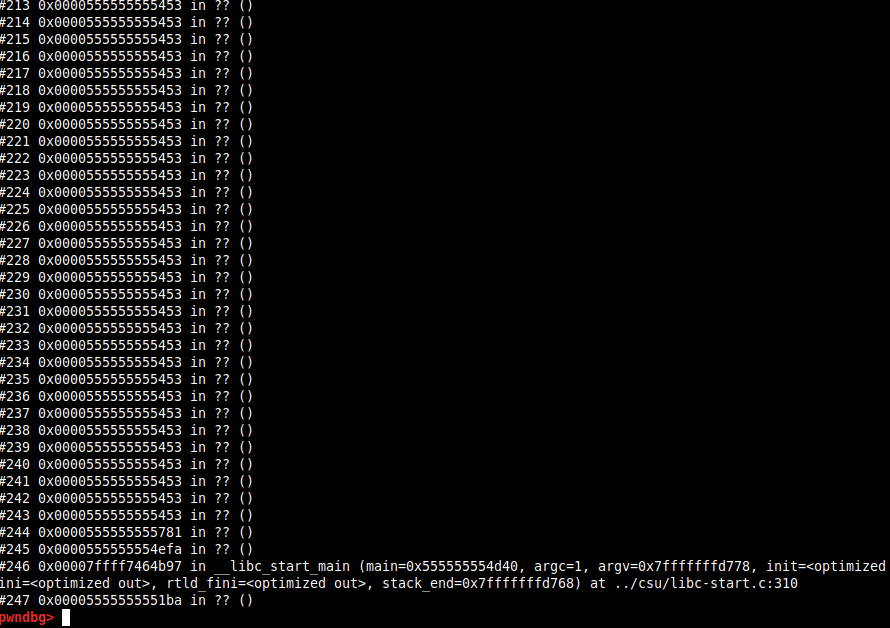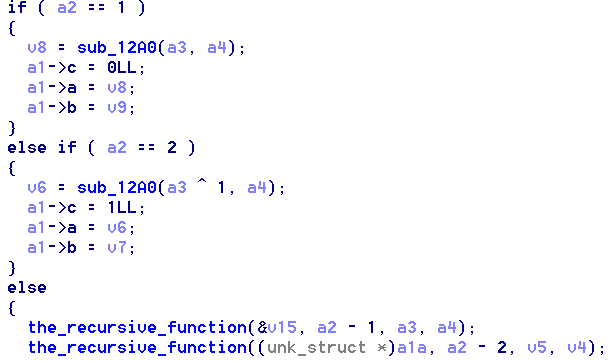# F-hash Writeup – Volga CTF 2020

Points: 250

Solves: 43

## The binary

We are given a C++ binary. Just running the binary gives you the flag, but it will “never” end. Our goal is to optimize it.

By looking at the backtrace in gdb we can see that function `sub_13b0` is being called recursively.After reversing it the minimal amount, I figured out that the function prototype is `void sub_13b0(res_struct *a1, int a2, int64_t a3, int64_t a4)`, where `res_struct` is:

``````struct res_struct {
int64_t a;
int64_t b;
int64_t c;
};
``````

We can also see that if `a2 == 1` or `a2 == 2` the recursion stops (base cases).## Solution

My idea was to memoize the results of the recursive function and replace `a1` with the cached result when a call with the same input (the combination of `a2`, `a3` and `a4`) occurs.

I didn’t want to reverse the function completely and implement it in python and so I decided to use frida to dynamically hook the function and do the memoization.

My first idea was to replace the function body by doing the following:

• new input: call the original function and cache the result
• cached input: write the cached result in `a1` and return immediately

Just as a POC this was the code I started with:

``````// frida --no-pause --runtime=v8 -l frida_trace.js ./f-hash

var recursive_func_ptr = ptr(f_hash_addr + 0x13b0);
var recursive_func = new NativeFunction(recursive_func_ptr, 'void', ['pointer', 'int64', 'int64', 'int64']);

Interceptor.replace(recursive_func_ptr, new NativeCallback(function (a1, a2, a3, a4) {
console.log(" the_recursive_function(a1: " + a1 + ", a2: " + a2 + ", a3: " + a3 + ", a4: " + a4 + ")");

// Call the original function
recursive_func(a1, a2, a3, a4)

}, 'void', ['pointer', 'int64', 'int64', 'int64']));
``````

The problem was that when I call the original function, its recursive calls won’t be hooked, and so it will just run forever as before. I didn’t come up with any way to do this by replacing the body.

My next idea was to use `OnEnter`, `OnLeave` and some hackery to get the job done:

• In the `OnEnter` hook:
• new input: do nothing
• cached input: make `a2 = 1` so that we hit a recursion base case (avoiding further recursion)
• In the `OnLeave` hook:
• new input: memoize the result
• cached input: replace the `a1` struct with the cached result (because we changed `a2` in the `onEnter` hook and so the result is wrong)

It was a bit of hacky solution but it works. The script:

``````// frida --no-pause --runtime=v8 -l frida_trace.js ./f-hash

// ====================
// UTILS
// ====================
function deref64(i) {
}

function p(s) {
console.log(s);
}

// ====================
// Hooks
// ====================
// Intercepts the recursive function
var mem = new Object();

function hash_args(args) {
return args + "_" + args + "_" + args;
};

var recursive_func_ptr = ptr(f_hash_addr + 0x13b0);

Interceptor.attach(recursive_func_ptr, {
onEnter: function(args) {
this.a1 = parseInt(args);
this.hash = hash_args(args);

if (typeof mem[this.hash] !== 'undefined') {
// Recursion stoppage case (we will replace the result in the OnLeave hook)
args = ptr(1);
}
},

onLeave: function(retval) {
if (typeof mem[this.hash] === 'undefined') {
// We have never seen this.. Memoize!
mem[this.hash] = [deref64(this.a1), deref64(this.a1+8), deref64(this.a1+16)];
} else {
// Just replace the fake result with the memoized one (because we made it run the recursion stoppage case)
var prev_results = mem[this.hash]
ptr(this.a1).writeU64(prev_results)
ptr(this.a1+8).writeU64(prev_results)
ptr(this.a1+16).writeU64(prev_results)
}
}
});
``````